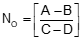### Article Index

This chapter explains several curve and tangent line-curve fitting situations.  They can be used with other COGO tools to construct complex curvilinear traverses having to fit specific mathematical conditions.

### A. Fitting arc through three points

Three non-linear points define a circular arc, Figure I-1Figure I-1 Three point arc

If the coordinates of the three points (1, 2, and 3) are known, the arc radius (R) and radius point (O) coordinates can be determined.

Equations I-1 and I-2 are used to compute the radius point coordinates.Equation I-1Equation I-2

The coefficients for Equation I-1 are:Equation I-3Equation I-4Equation I-5Equation I-6

Once the radius point coordinates are determined, the arc radius can be computed from Equation I-7 .Equation I-7

Point i is any of the three points used to define the arc.